# Clustering Using K-means Algorithm

This article explains K-means algorithm in an easy way. I’d like to start with an example to understand the objective of this powerful technique in machine learning before getting into the algorithm, which is quite simple.comments

By Firdaouss Doukkali, Machine Learning Engineer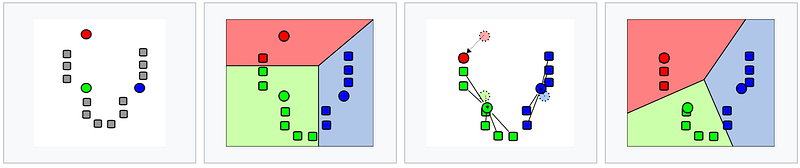k-means demonstration from Wikipedia

This article explains K-means algorithm in an easy way. I’d like to start with an example to understand the objective of this powerful technique in machine learning before getting into the algorithm, which is quite simple.

So imagine you have a set of numerical data of cancer tumors in 4 different stages from 1 to 4, and you need to study all the tumors in each stage. However, you have no idea how to identify the tumors that are at the same stage because nobody had the time to label the entire set of features (most data in the world are unlabeled). In this case, you need K-means algorithm because it works on unlabeled numerical data and it will automatically and quickly group them together into 4 clusters.

For this example, we chose k=4 because, we already know, we have 4 tumors’ stages, but if we want to cluster them based on their structure, growth speed, or growth type, then maybe k will be different than 4.

If you don’t know how many groups you want, it’s problematical, because K-means needs a specific number k of clusters in order to use it. So, the first lesson, whenever, you have to optimize and solve a problem, you should know your data and on what basis you want to group them. Then, you will be able to determine the number of clusters you need.

But, most of the time, we really have no idea what the right number of clusters is, so no worries, there is a solution for it, that we will discuss it later in this post.

### k-means Algorithm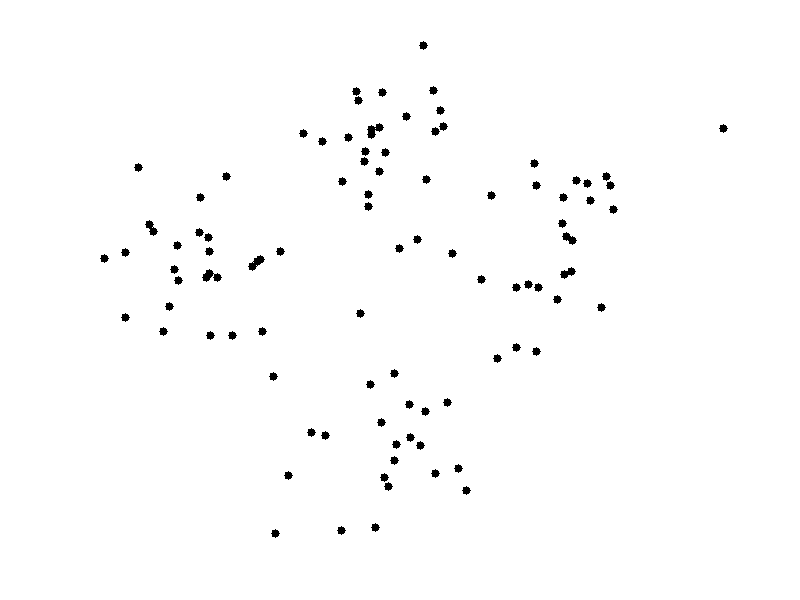from K-means clustering, credit to Andrey A. Shabalin

As, you can see, k-means algorithm is composed of 3 steps:

Step 1: Initialization

The first thing k-means does, is randomly choose K examples (data points) from the dataset (the 4 green points) as initial centroids and that’s simply because it does not know yet where the center of each cluster is. (a centroid is the center of a cluster).

Step 2: Cluster Assignment

Then, all the data points that are the closest (similar) to a centroid will create a cluster. If we’re using the Euclidean distance between data points and every centroid, a straight line is drawn between two centroids, then a perpendicular bisector (boundary line) divides this line into two clusters.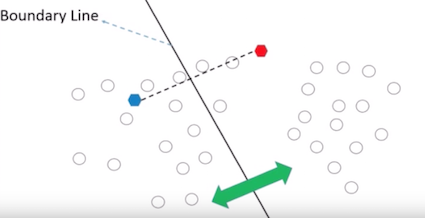from Introduction to Clustering and K-means Algorithm

Step 3: Move the centroid

Now, we have new clusters, that need centers. A centroid’s new value is going to be the mean of all the examples in a cluster.

We’ll keep repeating step 2 and 3 until the centroids stop moving, in other words, K-means algorithm is converged.

Here is the k-means algorithm: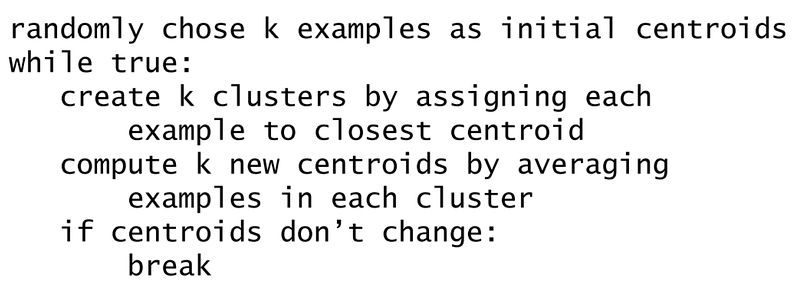from MIT 6.0002 lecture 12

K-means is a fast and efficient method, because the complexity of one iteration is k*n*d where k (number of clusters), n (number of examples), and d (time of computing the Euclidian distance between 2 points).

Then, how do we choose the number of clusters k?

In case, it is not clear, we try different values of k, we evaluate them and we choose the best k value.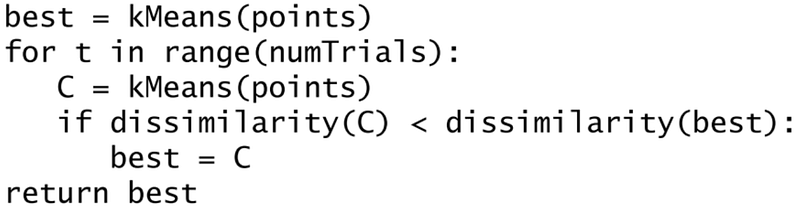from MIT 6.0002 lecture 12

Dissimilarity(C) is the sum of all the variabilities of k clusters

Variability is the sum of all Euclidean distances between the centroid and each example in the cluster.

Or you can take a small subset of your data, apply hierarchical clustering on it (it’s a slow clustering algorithm) to get an understanding of the data structure before choosing k by hand.

Unlucky centroids: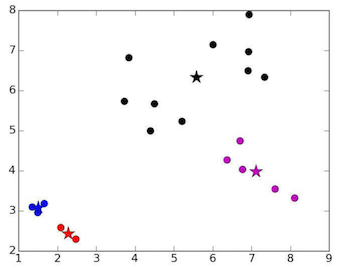The blue and red stars are unlucky centroids :( . From MIT 6.0002 lecture 12

Choosing poorly the random initial centroids will take longer to converge or get stuck on local optima which may result in bad clustering. in the picture above, the blue and red stars are unlucky centroids.

There are two solutions:

• Distribute them over the space.
• Try different sets of random centroids, and choose the best set.Lecture Slides and Files | Introduction to Computational Thinking and Data Science | Electrical…
This section includes lecture notes for the class, including associated files.

K-Means Algorithm - Stanford University | Coursera
Machine learning is the science of getting computers to act without being explicitly programmed. In the past decade…

Bio: Firdaouss Doukkali is a Machine Learning Engineer and Chief Unicorn Scientist. Global Shaper at World Economic Forum. English, French, German, Arabic, and Japanese speaker.

Original. Reposted with permission.

Related: